Technology Articles

# Code Search: a Closer Look at Akvelon’s Source Code Search Engine

As a developer or engineer, finding the right code snippet for a particular task can be a daunting challenge, especially with the vast array of libraries, frameworks, and codebases available on the internet.

At Akvelon, we understand the challenges that developers face in finding the right code snippet, which is why we have developed a Code Search service that helps developers quickly and easily search through multiple GitHub databases to find the code they need.

• How we built a search engine for the source code
• What is BERT and how to measure the meaning distance between two sentences using BERT
• UniXCoder: a BERT-like model for both source code and natural language
• How to perform fast search in a multidimensional vector space using Milvus
• How our Code Search service works

## Leveraging Machine Learning to Build a Source Code Search Engine

In order to build a source code search engine, we first need to implement an algorithm that estimates the relevance of a code snippet to the user request.

### BERT

Imagine we have two sentences. How do we measure the similarity of their meanings? We need to turn them into vectors (embeddings) and then calculate a certain distance metric (such as euclidean distance or cosine similarity).

Historically, there have been several approaches to the textual embedding problem, such as TF-IDF or Word2Vec. However, the idea that currently performs the best is BERT.

BERT is a transformer-based neural network that was introduced in 2018. It receives the sentence as a sequence of tokens and then returns the vector representation for each token that indicates its contextual meaning. In order to calculate the vector representation for the entire sentence, a process called “pooling” is performed: for each dimension, it averages the dimension values of all tokens in the sentence.

There are a lot of BERT models that vary in architecture, training process, training data, and assumed tokenization. For this project, we used the Sentence Transformers Python library that provides access to the all-mpnet-base-v2 model (you can check out other models here). This version of BERT was trained to measure the meaning distance between sentences specifically.

The Sentence_Transformers library is pretty straightforward to use: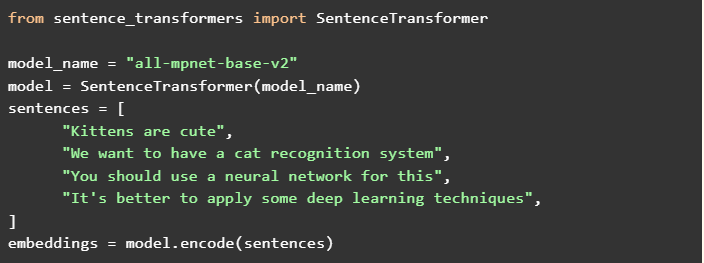If we calculate pairwise the cosine similarities between embeddings of our sentences, we get the following similarity matrix: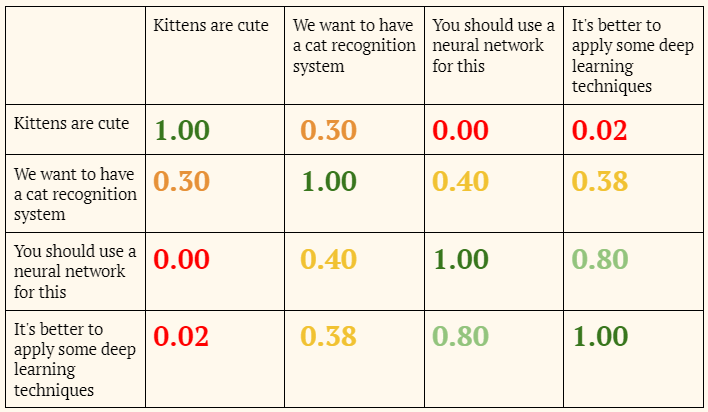These results make a lot of sense:

• Pairs like (“Kittens are cute”; “You must use a neural network for this”) have nothing in common, so their similarity is close to 0. Cosine similarity ranges from -1 to 1: two proportional vectors have a cosine similarity of 1, two orthogonal vectors have a similarity of 0, and two opposite vectors have a similarity of -1.
• Pairs like (“Kittens are cute”; “We want to have a cat recognition system”) or (“We want to have a cat recognition system”; “You should use a neural network for this”) talk about similar topics, so their similarity is medium.
• “You should use a neural network for this” and “It's better to apply some deep learning techniques” are almost synonymous, so the cosine similarity between them is quite high.

For each code snippet we must:

• Parse the signature of it. For example, the signature might looks like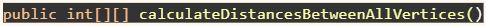• Normalize the signature: Convert it into the form that looks more similar to natural language.  At this step, we process all identifiers written in camelCase, replace _ characters with spaces and bring the text to a lower register. For example, “public int[][] calculateDistancesBetweenAllVertices()” becomes “public int[][] calculate distances between all vertices()”
• Calculate the BERT embedding of the normalized signature

The cosine similarity of two normalized vectors (euclidean norm = 1) is the same as their dot product. For this reason, we normalize all our embeddings and then use the dot product as a similarity metric.

### UniXcoder

However, just using only BERT similarities between the snippet signature and the user request is not enough. The method can have a name that is very similar to the request but still be completely irrelevant. We need to understand the code itself somehow.

One idea would be to calculate the BERT embedding of the entire snippet code. It will work, but BERT is designed to handle the natural language, not the source code.

Can we have something like BERT that can handle both English and programming languages? Yes we can! It’s exactly what UniXcoder does.

UniXcoder is a multimodal BERT-like model. If you embed a piece of code with it and its corresponding description in English, these embeddings will be similar.

UniXCoder treats the source code as a syntax tree rather than a sequence of tokens. UniXCoder developers state in their paper that UniXcoder outperforms other models at different code understanding tasks.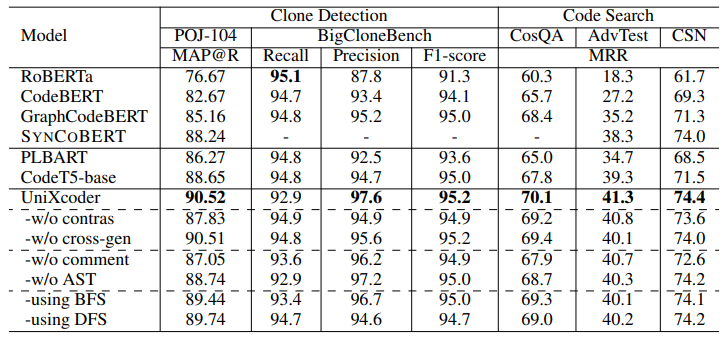### Embedding Ensemble

We can combine similarities obtained by different embeddings in order to improve the search.

Let U be the cosine similarity between the snippet UniXcoder embedding and the user request UniXcoder embedding, and let B be the cosine similarity between the snippet signature BERT embedding and the user request BERT embedding.

How can we tell if a certain S(U, B) formula is good or not? We need to quantify the search quality for a given formula. We have developed a GPT3-based solution that receives the snippet and the search request and then produces a single relevance value, from 0 to 9. This solution involves a giant neural network which is only available through the commercial API, which makes it unusable in the production scenario. However, we can run it a limited number of times offline. We used this model to derive the optional S(U, B) formula.

Assuming we have given a S(U, B) and a search request,  we can find the top 10 snippets by their relevance to the request. Then, for each of the top snippets we estimate the relevance R with our neural network. We calculate the search quality score as a sum of R/k where k is the rank of the snippet (from 1 to 10).

We came up with several search requests. For each possible S(U, B) formula we estimate the search quality on all of these requests and rank formulas by the average search quality.

The formula which leads to the best search results looks like: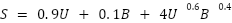### Backend

We have gathered a large amount of Java source code from Github and then parsed it with the tree-sitter library to get method snippets. As a result, we have more than 1,300,000 Java snippets in the Postgres database.

For each snippet, we have calculated the BERT embedding of the signature and the UniXcoder embedding of the code and stored these embeddings in the database as well.

At runtime, we calculate BERT and UniXcoder embeddings of the user search query. Our goal now is to obtain an array of snippets from the database that have the largest relevance score, which we define with our formula S = 0.9U + 0.1B + 4U0.6B0.4. How can we do that quickly if there are more than a million snippets in the database? That’s where Milvus comes into play.

### Milvus

Milvus is a tool for performing fast searches in a multidimensional vector space. It works in the following fashion:

1. We take a large number of vectors and create a Mulvis “collection” out of them
2. We build a Milvus “index” file of that collection. We can have many different indexes for the same collection. Indexes can differ by the type, similarity metric used, and some other settings
3. Given a random new vector and the index file, Milvus can quickly return N vectors from the collection that are the closest to our vector

In order to use Milvus from Python, we utilized the pyMilvus library.

The search speed depends on the collection size and index settings. For our purposes, we used IVF_SQ8 index with IP (inner product) similarity metric.

### Search

Let’s take a look at our formula again:We notice that the UniXcoder similarity affects the relevance score way more than the BERT similarity. Top snippets according to our formula can’t really have low UnixCoder similarity.

It means that if we ask Milvus to retrieve a modest number of snippets that have a high value of U, the resulting list will contain top snippets according to the entire formula.

We have built the Milvus collection and the index file on UniXcoder embedding only. Using this index, we retrieve 200 snippets that are closest to the user request by their UniXcoder embeddings. Then we sort these 200 snippets by their relevance value according to the formula (utilizing the BERT embedding of the request and BERT embeddings from the database) and present them to the user.

We have implemented the backend using FastAPI. FastAPI has a built-in swagger which allows us to not bother with the frontend and implement the UI on the backend entirely.

Backend Resources

It’s important to note that calculating both types of embeddings, creating a Milvus collection, and building an index is quite consuming in terms of computational resources. For example, on 2 CPUs with 8 GB of RAM each it would take about 4 days to generate all the UniXcoder embeddings using a single process.

In order to save time we have implemented several separate services and ran them in parallel.

### Results

Sum Array Elements

Let’s search for something simple first. Can it give us a code that sums elements of an array?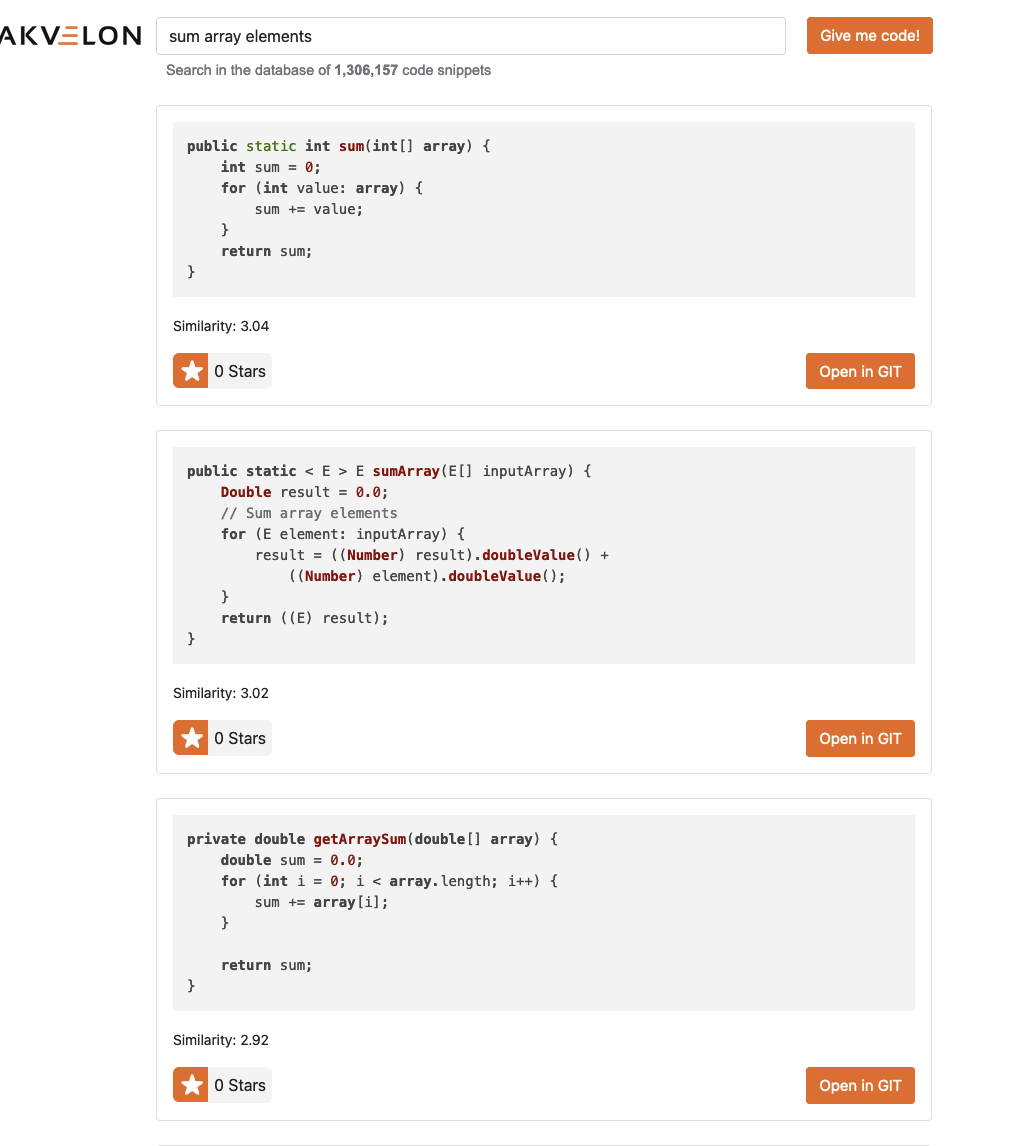The first result is what we were asking for: the method just sums the elements of an integer array using a foreach loop.

The second result is a bit more abstract: it uses Java generics for the array, assuming it can have any numerical type.

The third snippet is very similar to the first one, but it receives a double array and uses the regular for loop.

Quick Sort

Let's try something from the algorithmic scope. Would our system be able to find an implementation of the quick sort algorithm?Yes, an implementation has been found. However, the first snippet references an unknown method partition

How can we find the implementation of this partition method? Easy, we hit the “Open in GIT” button and voila! We were sent to the Github page and now we can dive into this specific implementation.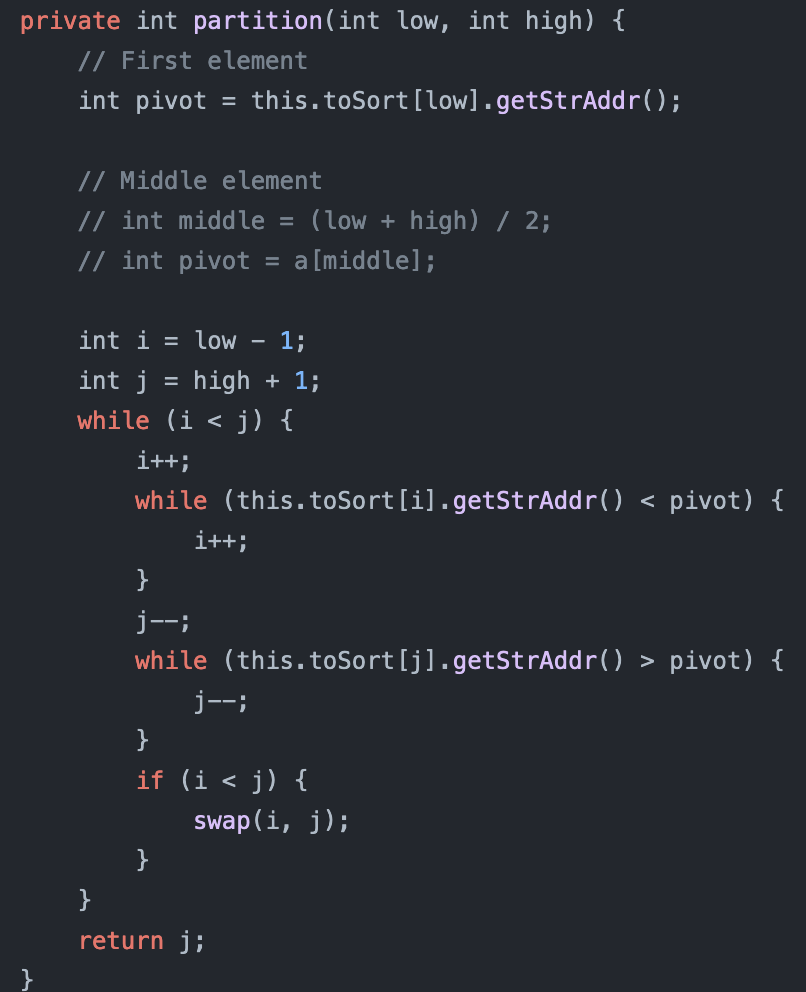Base64 Encoding

Let's ask something very specific. How to encode the text in the Base64 format?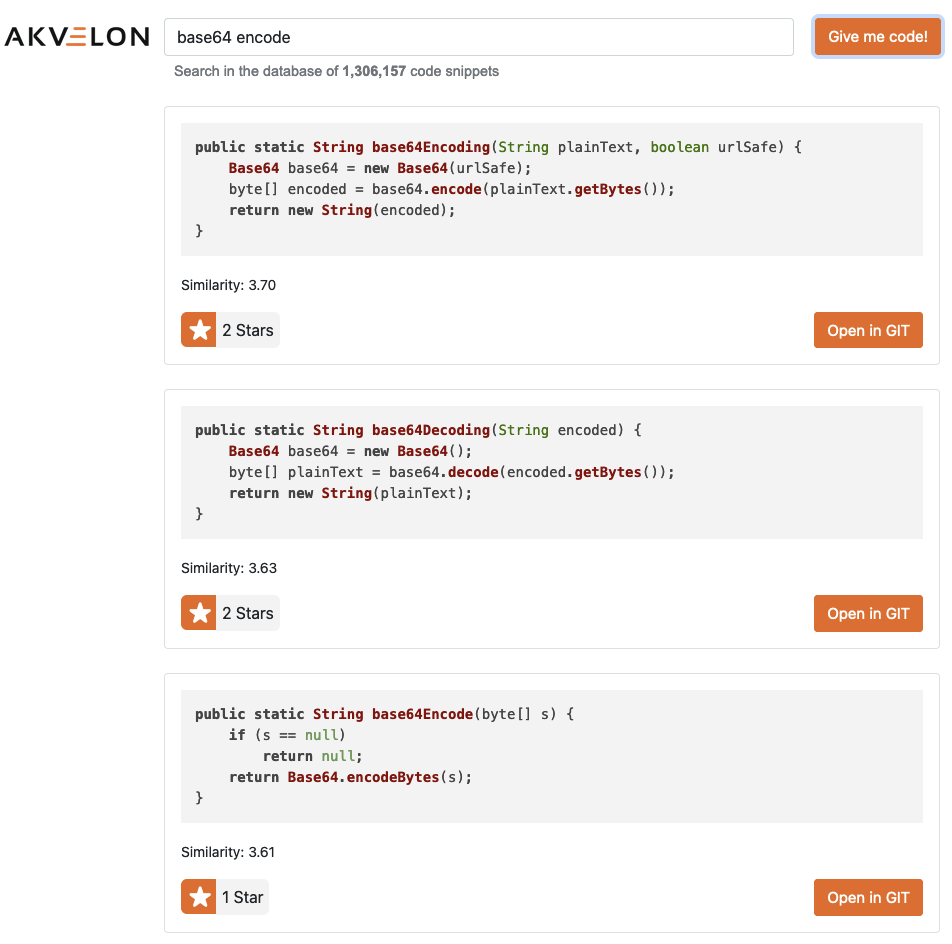Yay! Not only did it give us the expected result, but it also found a method that decodes the result back.

Android Toast

Let's make a platform-specific request. In Android, there is a thing called “toast”: a small textual notification that appears on the bottom of the screen for a while and then disappears.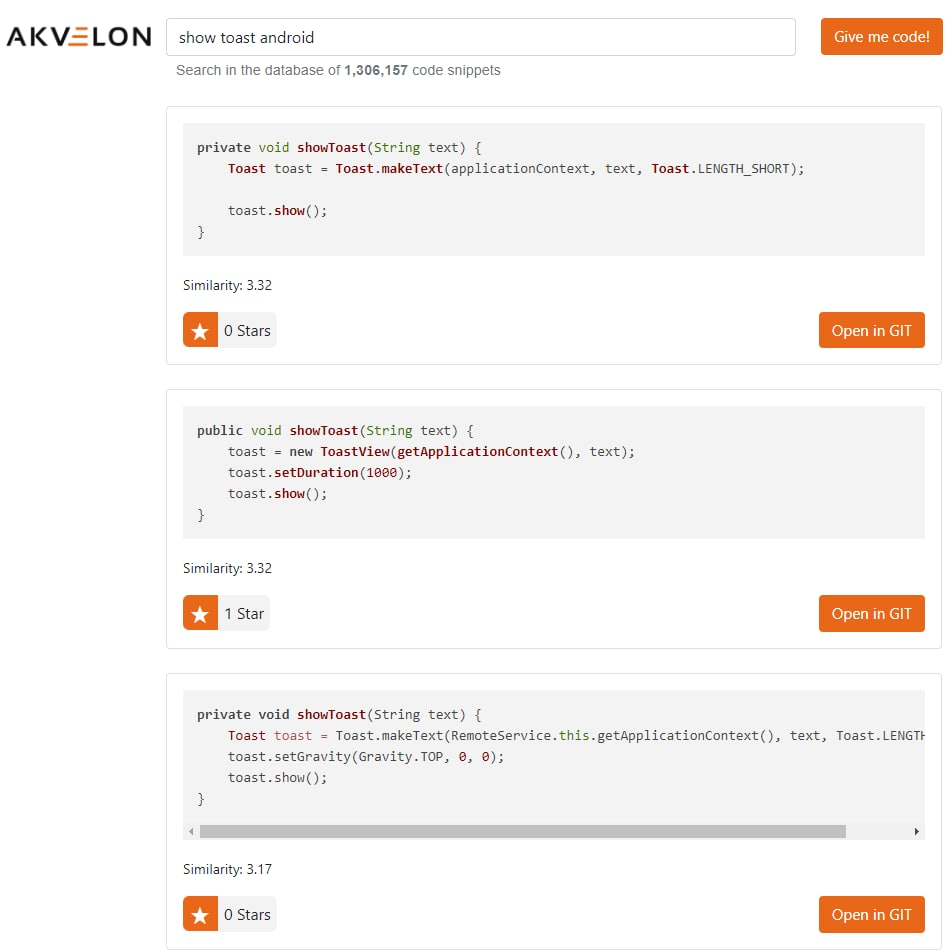It does work out without any problem!

Tree Height

Let’s assume we have some kind of tree structure in our code. Can we find a method that computes the height of the tree?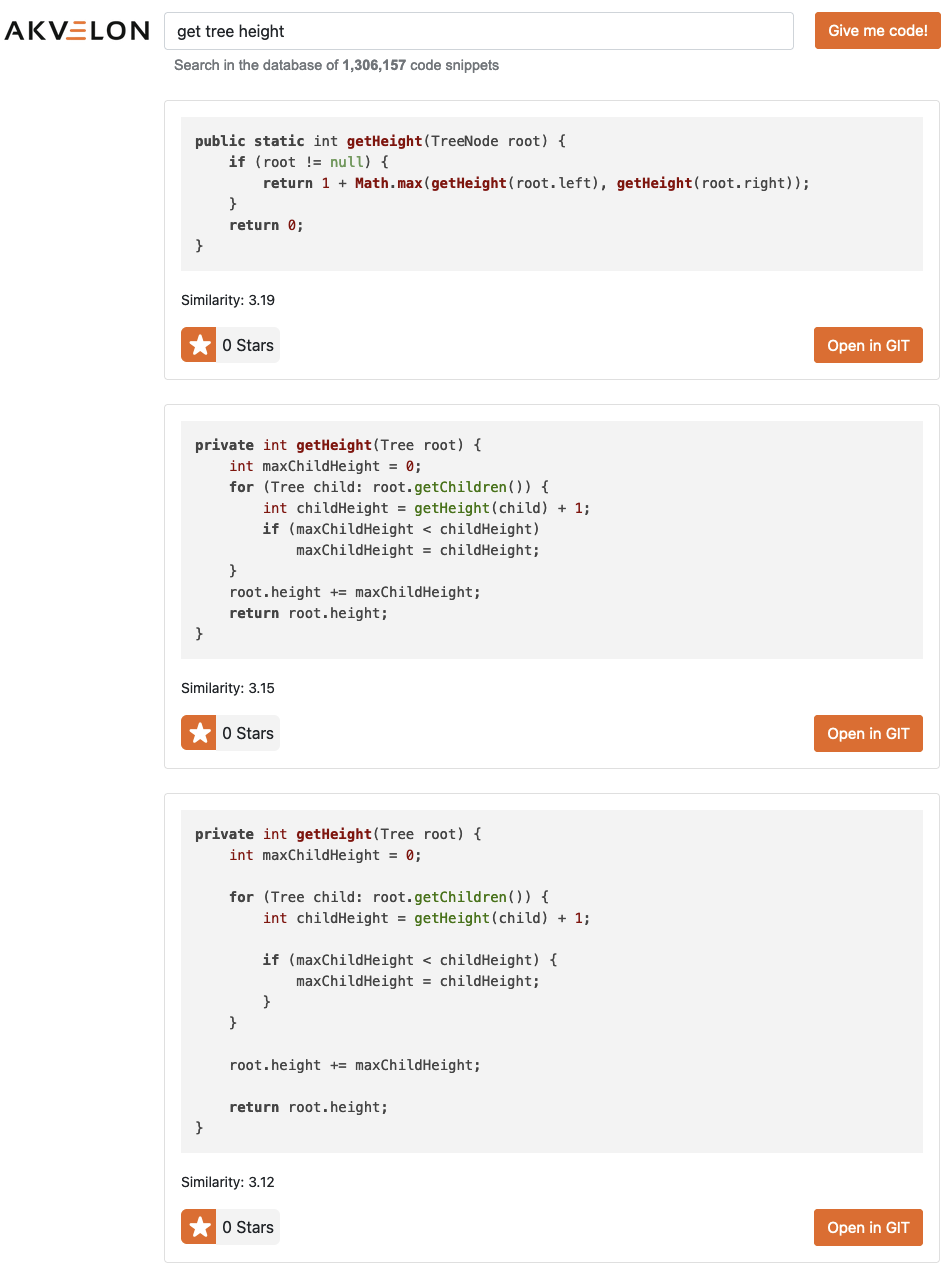The first result is a very short recursive algorithm that does the job.

Get Current Time

If we forget the specific way to handle dates and times in Java, we can ask the search engine: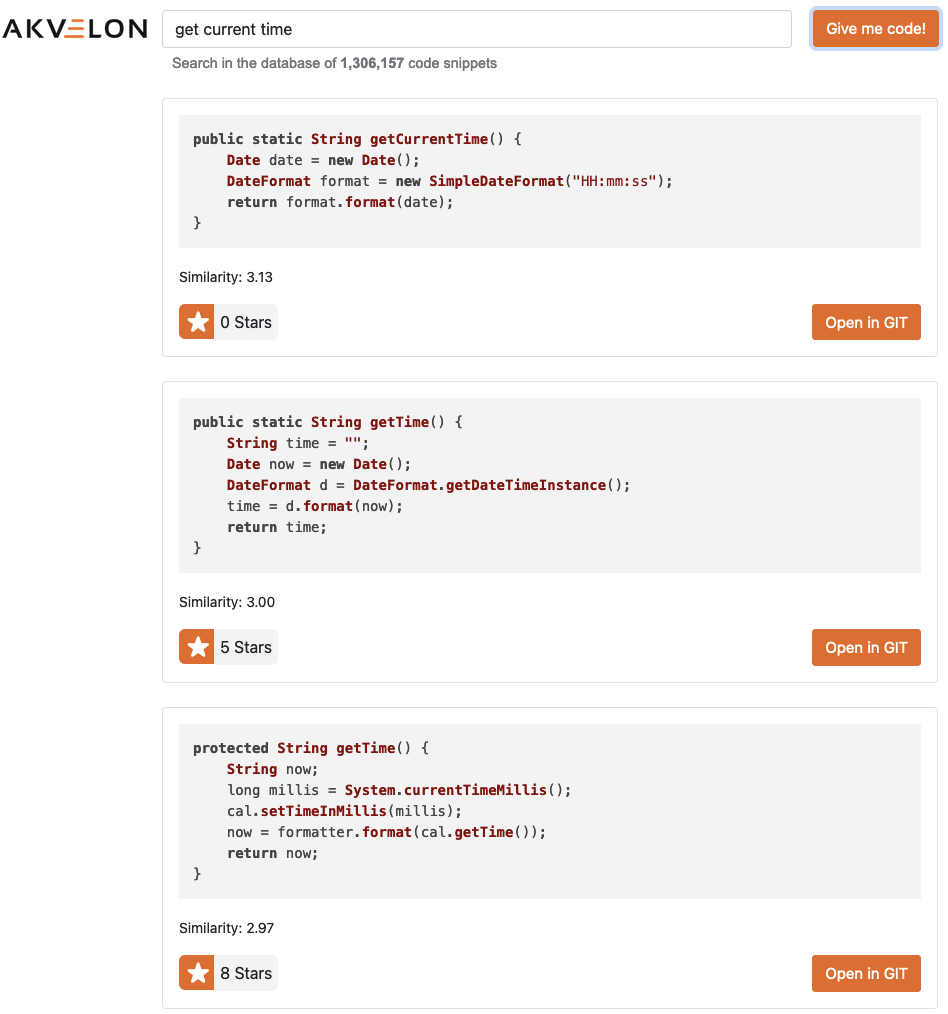It does work out - all the result snippets return the current time.

### Conclusion

With features such as code highlighting and code repository licenses, the search process is made even more convenient. Our team has experimented with different solutions and found the best way to implement our idea, resulting in a user-friendly tool that saves specialists both time and effort when searching for code snippets.

Try it out today and see how much more efficient your coding process can be. Access the application here.

### Dmitrii Lukianov

Data Scientist at Akvelon

### Natalia Baranova

Software Development Engineer at Akvelon